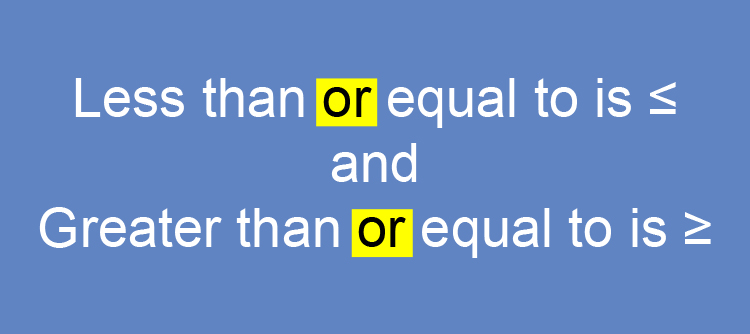# Inequalities and equal numbers

8>=8 reads as

8  is greater than OR equal to 8

Because:The highlighted 'or' is very important.‘OR’ and oars are very important.

Examples

1.  True or false 9>3 ?

Answer: 9 is greater than 3, so this is True.

2.  True or false 9>=3 ?

Read as 9 is greater than or equal to 3. This is true.

NOTE:

It only needs to be one of these.

3.  True or false 3 < 6 ?

Answer: 3 is less than 6 so this is true.

4.  True or false

{6(3-1)+3}/(2times2+1}>=2

{6(2)+3}/(4+1}>=2

{12+3}/(5)>=2

15/(5)>=2

3>=2

Read as 3 is greater than or equal to 2 which is true.

5.  True or false 8 ≥ 8 ?

Read as 8 is greater than OR equal to 8

This statement is true.

6.  True or false  9times4-12<=2times12 ?

So work out each side

36-12<=24

24<=24

Read as 24 is less than OR equal to 24.

This statement is true.